# US20100115359A1 - Method of encoding/decoding using low density check code matrix - Google Patents

Method of encoding/decoding using low density check code matrix Download PDF

## Info

Publication number
US20100115359A1
US20100115359A1 US12/373,726 US37372607A US2010115359A1 US 20100115359 A1 US20100115359 A1 US 20100115359A1 US 37372607 A US37372607 A US 37372607A US 2010115359 A1 US2010115359 A1 US 2010115359A1
Authority
US
United States
Prior art keywords
sub
matrix
parity
method
part
Prior art date
Legal status (The legal status is an assumption and is not a legal conclusion. Google has not performed a legal analysis and makes no representation as to the accuracy of the status listed.)
Granted
Application number
US12/373,726
Other versions
US8201046B2 (en
Inventor
Ji Wook Chung
Min Seok Oh
Ki Hyoung Cho
Ji Ae Seok
Young Seob Lee
So Yeon Kim
Current Assignee (The listed assignees may be inaccurate. Google has not performed a legal analysis and makes no representation or warranty as to the accuracy of the list.)
LG Electronics Inc
Original Assignee
LG Electronics Inc
Priority date (The priority date is an assumption and is not a legal conclusion. Google has not performed a legal analysis and makes no representation as to the accuracy of the date listed.)
Filing date
Publication date
Priority to KR10-2006-0066465 priority Critical
Priority to KR1020060066465A priority patent/KR101154995B1/en
Application filed by LG Electronics Inc filed Critical LG Electronics Inc
Priority to PCT/KR2007/003416 priority patent/WO2008007927A2/en
Assigned to LG ELECTRONICS INC. reassignment LG ELECTRONICS INC. ASSIGNMENT OF ASSIGNORS INTEREST (SEE DOCUMENT FOR DETAILS). Assignors: KIM, SO YEON, OH, MIN SECK, CHUNG, JI WOOK, SEOK, JI AE, LEE, YOUNG SEOB, CHO, KI HYOUNG
Publication of US20100115359A1 publication Critical patent/US20100115359A1/en
Assigned to LG ELECTRONICS INC. reassignment LG ELECTRONICS INC. CORRECTIVE ASSIGNMENT TO CORRECT THE NAME OF SECOND ASSIGNOR AND THE EXECUTION DATES OF THIRD AND FIFTH ASSIGNORS PREVIOUSLY RECORDED ON REEL 023576 FRAME 0361. ASSIGNOR(S) HEREBY CONFIRMS THE SECOND ASSIGNOR'S NAME IS MIN SEOK OH; THE EXECUTION DATES FOR KI HYOUNG CHO IS OCT-27-09 AND FOR YOUNG SEOB LEE IS OCT-26-09. Assignors: CHUN, JI WOOK, KIM, SO YEON, OH, MIN SEOK, SEOK, JI AE, CHO, KI HYOUNG, LEE, YOUNG SEOB
Publication of US8201046B2 publication Critical patent/US8201046B2/en
Application granted granted Critical
Application status is Active legal-status Critical
Adjusted expiration legal-status Critical

• 239000011159 matrix materials Substances 0 Abstract Claims Description Title 385
• 230000000875 corresponding Effects 0 Claims Description 46
• 238000010295 mobile communication Methods 0 Claims Description 9
• 238000007792 addition Methods 0 Description 1
• 230000003935 attention Effects 0 Description 2
• 230000033590 base-excision repair Effects 0 Description 2
• 238000004422 calculation algorithm Methods 0 Description 2
• 238000004364 calculation methods Methods 0 Description 36
• 238000004891 communication Methods 0 Description 7
• 230000001276 controlling effects Effects 0 Description 1
• 230000003247 decreasing Effects 0 Description 1
• 230000003467 diminishing Effects 0 Description 1
• 238000005562 fading Methods 0 Description 2
• 230000001976 improved Effects 0 Description 3
• 230000001965 increased Effects 0 Description 4
• 230000015654 memory Effects 0 Description 4
• 238000000034 methods Methods 0 Description 16
• 238000006011 modification Methods 0 Description 1
• 230000004048 modification Effects 0 Description 1
• 230000000051 modifying Effects 0 Description 6
• 230000003287 optical Effects 0 Description 1
• 239000000047 products Substances 0 Description 3
• 230000002829 reduced Effects 0 Description 2
• 230000001603 reducing Effects 0 Description 2
• 238000006722 reduction reaction Methods 0 Description 2
• 230000003252 repetitive Effects 0 Description 3
• 230000004044 response Effects 0 Description 4
• 230000001702 transmitter Effects 0 Description 31

## Images

•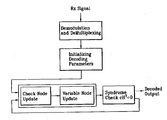•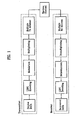•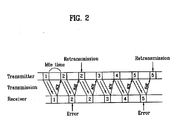•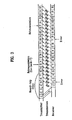•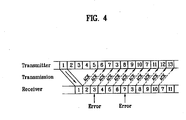•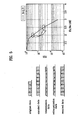•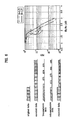••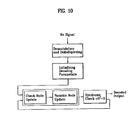•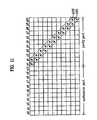•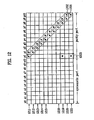•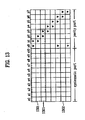•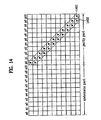•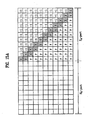•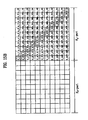•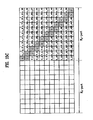•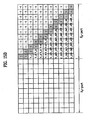•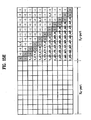•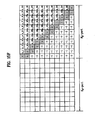•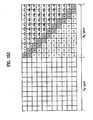•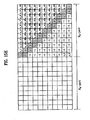•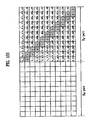•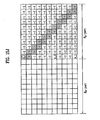•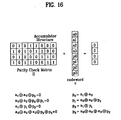•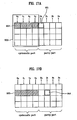•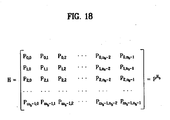•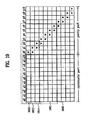•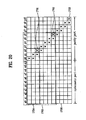•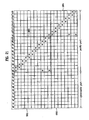•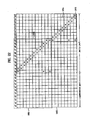••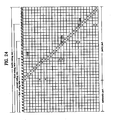•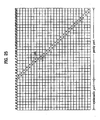••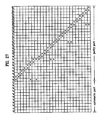•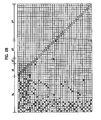•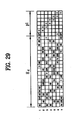•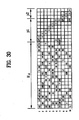••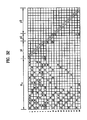•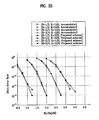•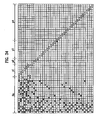•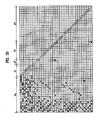•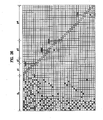## Classifications

• HELECTRICITY
• H03BASIC ELECTRONIC CIRCUITRY
• H03MCODING; DECODING; CODE CONVERSION IN GENERAL
• H03M13/00Coding, decoding or code conversion, for error detection or error correction; Coding theory basic assumptions; Coding bounds; Error probability evaluation methods; Channel models; Simulation or testing of codes
• H03M13/03Error detection or forward error correction by redundancy in data representation, i.e. code words containing more digits than the source words
• H03M13/05Error detection or forward error correction by redundancy in data representation, i.e. code words containing more digits than the source words using block codes, i.e. a predetermined number of check bits joined to a predetermined number of information bits
• H03M13/11Error detection or forward error correction by redundancy in data representation, i.e. code words containing more digits than the source words using block codes, i.e. a predetermined number of check bits joined to a predetermined number of information bits using multiple parity bits
• H03M13/1102Codes on graphs and decoding on graphs, e.g. low-density parity check [LDPC] codes
• H03M13/1148Structural properties of the code parity-check or generator matrix
• H03M13/118Parity check matrix structured for simplifying encoding, e.g. by having a triangular or an approximate triangular structure
• H03M13/1185Parity check matrix structured for simplifying encoding, e.g. by having a triangular or an approximate triangular structure wherein the parity-check matrix comprises a part with a double-diagonal
• H03M13/1188Parity check matrix structured for simplifying encoding, e.g. by having a triangular or an approximate triangular structure wherein the parity-check matrix comprises a part with a double-diagonal wherein in the part with the double-diagonal at least one column has an odd column weight equal or greater than three
• HELECTRICITY
• H03BASIC ELECTRONIC CIRCUITRY
• H03MCODING; DECODING; CODE CONVERSION IN GENERAL
• H03M13/00Coding, decoding or code conversion, for error detection or error correction; Coding theory basic assumptions; Coding bounds; Error probability evaluation methods; Channel models; Simulation or testing of codes
• H03M13/03Error detection or forward error correction by redundancy in data representation, i.e. code words containing more digits than the source words
• H03M13/05Error detection or forward error correction by redundancy in data representation, i.e. code words containing more digits than the source words using block codes, i.e. a predetermined number of check bits joined to a predetermined number of information bits
• H03M13/11Error detection or forward error correction by redundancy in data representation, i.e. code words containing more digits than the source words using block codes, i.e. a predetermined number of check bits joined to a predetermined number of information bits using multiple parity bits
• H03M13/1102Codes on graphs and decoding on graphs, e.g. low-density parity check [LDPC] codes
• HELECTRICITY
• H03BASIC ELECTRONIC CIRCUITRY
• H03MCODING; DECODING; CODE CONVERSION IN GENERAL
• H03M13/00Coding, decoding or code conversion, for error detection or error correction; Coding theory basic assumptions; Coding bounds; Error probability evaluation methods; Channel models; Simulation or testing of codes
• H03M13/03Error detection or forward error correction by redundancy in data representation, i.e. code words containing more digits than the source words
• H03M13/05Error detection or forward error correction by redundancy in data representation, i.e. code words containing more digits than the source words using block codes, i.e. a predetermined number of check bits joined to a predetermined number of information bits
• H03M13/11Error detection or forward error correction by redundancy in data representation, i.e. code words containing more digits than the source words using block codes, i.e. a predetermined number of check bits joined to a predetermined number of information bits using multiple parity bits
• H03M13/1102Codes on graphs and decoding on graphs, e.g. low-density parity check [LDPC] codes
• H03M13/1148Structural properties of the code parity-check or generator matrix
• H03M13/118Parity check matrix structured for simplifying encoding, e.g. by having a triangular or an approximate triangular structure
• H03M13/1185Parity check matrix structured for simplifying encoding, e.g. by having a triangular or an approximate triangular structure wherein the parity-check matrix comprises a part with a double-diagonal
• HELECTRICITY
• H03BASIC ELECTRONIC CIRCUITRY
• H03MCODING; DECODING; CODE CONVERSION IN GENERAL
• H03M13/00Coding, decoding or code conversion, for error detection or error correction; Coding theory basic assumptions; Coding bounds; Error probability evaluation methods; Channel models; Simulation or testing of codes
• H03M13/63Joint error correction and other techniques
• H03M13/6306Error control coding in combination with Automatic Repeat reQuest [ARQ] and diversity transmission, e.g. coding schemes for the multiple transmission of the same information or the transmission of incremental redundancy
• HELECTRICITY
• H03BASIC ELECTRONIC CIRCUITRY
• H03MCODING; DECODING; CODE CONVERSION IN GENERAL
• H03M13/00Coding, decoding or code conversion, for error detection or error correction; Coding theory basic assumptions; Coding bounds; Error probability evaluation methods; Channel models; Simulation or testing of codes
• H03M13/63Joint error correction and other techniques
• H03M13/635Error control coding in combination with rate matching
• H03M13/6362Error control coding in combination with rate matching by puncturing
• H03M13/6368Error control coding in combination with rate matching by puncturing using rate compatible puncturing or complementary puncturing
• H03M13/6393Rate compatible low-density parity check [LDPC] codes
• HELECTRICITY
• H03BASIC ELECTRONIC CIRCUITRY
• H03MCODING; DECODING; CODE CONVERSION IN GENERAL
• H03M13/00Coding, decoding or code conversion, for error detection or error correction; Coding theory basic assumptions; Coding bounds; Error probability evaluation methods; Channel models; Simulation or testing of codes
• H03M13/65Purpose and implementation aspects
• H03M13/6522Intended application, e.g. transmission or communication standard
• H03M13/6544IEEE 802.16 (WIMAX and broadband wireless access)
• HELECTRICITY
• H04ELECTRIC COMMUNICATION TECHNIQUE
• H04LTRANSMISSION OF DIGITAL INFORMATION, e.g. TELEGRAPHIC COMMUNICATION
• H04L1/00Arrangements for detecting or preventing errors in the information received
• H04L1/004Arrangements for detecting or preventing errors in the information received by using forward error control
• H04L1/0056Systems characterized by the type of code used
• H04L1/0057Block codes
• HELECTRICITY
• H04ELECTRIC COMMUNICATION TECHNIQUE
• H04LTRANSMISSION OF DIGITAL INFORMATION, e.g. TELEGRAPHIC COMMUNICATION
• H04L1/00Arrangements for detecting or preventing errors in the information received
• H04L1/12Arrangements for detecting or preventing errors in the information received by using return channel
• H04L1/16Arrangements for detecting or preventing errors in the information received by using return channel in which the return channel carries supervisory signals, e.g. repetition request signals
• H04L1/18Automatic repetition systems, e.g. van Duuren system ; ARQ protocols
• H04L1/1806Go-back-N protocols

## Abstract

A method of encoding data using a parity check matrix is disclosed. The method of encoding data using a parity check matrix includes receiving information bit streams, and encoding the information bit streams using the parity check matrix which includes a systematic part and a parity part having a lower triangle type.

## Description

TECHNICAL FIELD
• The present invention relates to a method of encoding/decoding using a low density parity check (LDPC) code, and more particularly, to a method of encoding data using a parity check matrix.
• BACKGROUND ART
• FIG. 1 illustrates a structure of a mobile communication channel to which the present invention and the related art are applied. Hereinafter, the structure of the mobile communication channel will be described with reference to FIG. 1. A transmitter undergoes a channel coding procedure to transmit data without loss or distortion through a radio channel. Examples of the channel coding include convolutional coding, turbo coding, LDPC coding, etc. The data which has undergone the channel coding procedure can be transmitted to the radio channel as a single symbol which includes several bits. At this time, a procedure of mapping several bits to a single symbol is referred to as modulation.
• The modulated data is converted into a signal for multiple transmission through a multiplexing procedure or a multiple access method. Examples of the multiplexing procedure include CDM, TDM, FDM, etc. An example of OFDM (Orthogonal Frequency Division Multiplexing) is shown in FIG. 1. The signal which has undergone the multiplexing block is changed to a structure suitable for transmission to one or more multi-antennas, and then is transferred to a receiver through the radio channel. Fading and thermal noise occur in the transmitted data when the data passes through the radio channel. For this reason, distortion may occur in the data.
• The modulated data is transferred to the receiver through the radio channel. In this case, fading and thermal noise occur in the transmitted data, whereby distortion may occur therein. The receiver performs a series of procedures of the transmitter in reverse order after receiving the distorted data. The receiver performs demodulation to convert the data mapped to the symbol into a bit stream, undergoes channel decoding, and restores the distorted data to the original data.
• An apparatus of performing the channel coding stores a matrix H or a matrix G, wherein the matrix H is a parity check matrix used to generate parity bits to be added to input data (systematic bits), and the matrix G is a parity check generate matrix derived from the matrix H. In other words, the transmitter includes an encoder which generates parity bits through the input data and the matrix H or G. An apparatus of performing channel decoding checks whether the systematic bits are restored well, through operation of the received data (distorted systematic bits+parity bits) with the matrix H, and performs operation again if the systematic bits are failed to be restored.
• Examples of the modulation include BPSK (Binary Phase Shift Keying), QPSK (Quadrature Phase Shift Keying), 16-QAM (Quadrature Amplitude Modulation), 64-QAM, 256-QAM, etc. For example, 16-QAM maps the data stream which has undergone channel encoding during modulation to a single symbol in a unit of 4 bits. 16-QAM de-maps the single symbol received through the radio channel during demodulation to four bits.
• Meanwhile, according to the Go-Back-N method, the transmitter first transmits N number of data and receives ACK messages from the receiver in due order. FIG. 3 illustrates a case of N=7, wherein the number N of data which are transmitted without ACK message is referred to as a window size. If the transmitter receives the NACK message in response to the kth data, the transmitter sequentially transmits data starting from the kth data.
• FIG. 4 illustrates a Selective Repeat method. According to the Selective Repeat method, like the Go-Back-N method, the number N of data which are transmitted without ACK or NACK message is referred to as a window size, and retransmission is selectively performed for only data with NACK message.
• According to the aforementioned HARQ method, if retransmission is performed in the ARQ method, previously transmitted data is combined with retransmitted data to restore the data through FEC symbol. The HARQ method is classified into a chase combining method and an incremental redundancy method depending, on a combining method of the two data. According to the chase combining method, as shown in FIG. 5, the receiver combines transmission data with retransmission data to increase a receiving signal to noise ratio (SNR), thereby increasing a receiving success ratio of data at the receiver.
• Meanwhile, unlike the chase combining method, the incremental redundancy method (hereinafter, referred to as ‘IR method’) transmits some of encoded data, which have not been used for first transmission, during retransmission of the transmitter to decrease a coding rate of data received at the receiver, thereby increasing a receiving success ratio.
• Hereinafter, LDPC coding will be described. A concept of LDPC coding is as follows.
• A linear code can be described with the parity check generation matrix G and the parity check matrix H. A characteristic of the linear code is that the equation of HcT=0 is satisfied for every bit of a codeword ‘c’. As one of the linear code, the LDPC code which is recently paid attention to was proposed by Gallager in 1962 for the first time. One of the characteristics of the LDPC coding is that most of elements of the parity check matrix H are ‘0’ and the number of elements which are not ‘0’ is small compared to the codeword, so that repetitive decoding based on probability is possible. A parity check matrix H for the first proposed LDPC code was defined in a non-systematic form and each row and column of the parity check matrix were designed to equally have a small weight.
• In this case, the weight means the number of ‘1’ included in each row and column.
• The LDPC coding scheme has low decoding complexity since a density of elements which are not ‘0’ in the parity check matrix H is low. Further, decoding performance of the LDPC coding is superior to other coding schemes, which is adjacent to the theoretical limit of Shannon. However, the LDPC coding scheme could not be implemented with the hardware technique at the time of proposal by Gallegar, so that the LDPC coding scheme has not been paid attention to by the people for 30 years. A repetitive decoding scheme using graphs was developed in early 1980's and a couple of decoding algorithms for the LDPC code have been developed using the repetitive decoding scheme. One of them is a sum-product algorithm.
• Hereinafter, the characteristic of the LDPC coding will be described. The LDPC coding has a superior error correction capability, thereby improving communication speed and capacity. When combined with a multi-input and multi-output (MIMO) scheme, the LDPC coding can be applied to a high speed wireless LAN having a data transmission speed of several hundred Mbit/s, a high speed mobile communication system having a data transmission speed of one Mbit/s for a user moving at a speed of 250 km/h, and an optical communication system having a data transmission speed of 40 Gbit/s. In addition, the LDPC coding can enable a quantum encryption communication diminishing the number of retransmission on a communication path having low quality since transmission quality is improved due to its high error correction capability. Further, data packets having errors can be easily recovered due to the low complexity and superior loss compensation capability of the LDPC coding, so that contents having quality equal to that of TV can be transmitted through the Internet and the mobile communication system. 10G BASE-T transmission within a range of 100m which has been considered impossible earlier can be realized owing to wide application range and large capacity which are advantages of the LDPC coding. In addition, transmission capacity of a single satellite transmitter having 36 MHz bandwidth can be increased up to 80 Mbit/s which is 1.3 times of usual transmission capacity. With the above described advantages, the LDPC coding scheme is adopted as a next generation channel coding scheme in a communication system, like IEEE 802.16 or IEEE 802.11, etc.
• Hereinafter, a structured LDPC will be described.
• A parity check matrix H is used to use LDPC code, and includes most of elements 0 and some elements 1. Since the matrix H has a great size of 105 bits or greater, a large sized memory is required to express the matrix H. The structured LDPC expresses the elements of the matrix H used for LDPC encoding and decoding as a sub-block of a certain size as shown in FIG. 7. In IEEE 802.16e, the sub-block is identified by one integer index to decrease the size of the memory required to store the matrix H. Various kinds of matrixes could be the sub-block. For example, a permutation matrix of a certain size could be the sub-block.
• If the structured LDPC is used, one integer (i.e., index) is only needed to be stored in a specific memory instead of a certain sized matrix consisting of elements of 1 or 0. Accordingly, the size of the memory required to express the matrix H can be decreased.
• For example, if a size of a codeword reflected in the IEEE802.16e standard is 2304 and a coding rate is ⅔, a model matrix used for LDPC encoding/decoding is as shown in FIG. 8.
• As shown in FIG. 8, the structured LDPC matrix of IEEE802.16e consists of elements of −1, 0 and positive integers, wherein −1 represents a zero matrix of which elements are all 0, and 0 represents an identity matrix. The positive integers excluding −1 and 0 constitute a permutation matrix in which the identity matrix is shifted to the right by positive integers. In other words, if elements constituting a matrix are 3, it represents a permutation matrix in which the identity matrix is shifted to the right three times.
• FIG. 9 illustrates a method of expressing a matrix according to the aforementioned positive integers, i.e., shift numbers. It is assumed that a specific matrix H is identified by a structured matrix of 4×4 (i.e., sub-block). In this case, if the specific sub block is identified by 3, the sub-block becomes a matrix of FIG. 9. 0
• Hereinafter, LDPC encoding method will be described.
• According to a general LDPC encoding method, information bits are encoded by using a generation matrix G derived from an LDPC parity check matrix H. To derive the generation matrix G, the parity check matrix H is configured in the form of [PT:I] by using a Gaussian reduction method. Assuming that the number of the information bits is ‘k’ and a size of an encoded codeword is ‘n’, the ‘P’ is a matrix having ‘k’ number of rows and ‘(n−k)’ number of columns and the ‘I’ is an identity matrix having ‘k’ number of rows and columns.
• When the parity check matrix H is represented in the form of [PT:I], the generation matrix G has the form of [I:P]. The information bits of k bits to be encoded can be represented as a matrix ‘x’ having one row and ‘k’ number of columns. In this case, the codeword ‘c’ is represented in the form of the following equation.

• c=xG=[x:xP]  [Equation 1]
• In the above equation, x represents a systematic part, and xP represents a parity part.
• Meanwhile, if encoding is performed by the Gaussian Reduction method as above, since a calculation amount is increased, the form of the matrix H is designed in a specific structure so that a method of directly encoding data in the matrix H without deriving the matrix G is used. In other words, if the equation 1 is multiplied by HT by using a feature (i.e., GHT=0) that a product between the matrix G and a transpose HT for the matrix H, the following equation 2 can be obtained. The codeword ‘c’ can be obtained by adding a parity bit suitable for the equation 2 next to the information bit ‘x.’

• cHT=xGHT=[x:xP][PT:I]T=0  [Equation 2]
• Hereinafter, LDPC decoding method will be described.
• Data encoded in a communication system includes noise when passing through a radio channel of FIG. 1. A receiver represents a decoding procedure of data through a procedure as shown in FIG. 10. A decoding block of the receiver obtains an information bit ‘x’ from a receiving signal c′ having the encoded codeword ‘c’ added with noise by using the feature of cHT=0. In other words, assuming that the received codeword is c′, a value of c′HT is calculated. As a result, if the value of c′HT is 0, first k number of bits from c′ are determined as the information bit x. If the value of c′HT is not 0, c′ which satisfies c′HT of 0 is searched by using a decoding method of a sum-product algorithm through a graph, thereby restoring the information bit x.
• Hereinafter, a coding rate of LDPC coding will be described.
• Generally, when the size of the information bit is k and the size of the codeword which is actually transmitted is n, a coding rate (R) is as follows.

• R=k/n  [Equation 3]
• When the matrix H necessary for LDPC encoding and decoding has a row size of m and a column size of n, a coding rate is as follows.

• R=1−m/n  [Equation 4]
• As described above, since the related art LDPC code is encoded and decoded by the matrix H, the structure of the matrix H is very important. In other words, since encoding and decoding performance is greatly affected by the structure of the matrix H, design of the matrix H is more important than anything else.
• The related art LDPC code has a problem in performing retransmission in accordance with the IR method. Although the IR method is a retransmission method which uses the correlation between the previously transmitted parity part and the retransmitted parity part, the correlation between the previously transmitted parity part and the retransmitted parity part does not exist if encoding is performed using the related art LDPC code. Accordingly, to apply the related art LDPC code to the IR method, modification of the related art LDPC code is needed.
• DISCLOSURE OF THE INVENTION
• Accordingly, the present invention is suggested to substantially obviate one or more problems due to limitations and disadvantages of the related art. An object of the present invention is to provide a method of encoding data by LDPC code which is applicable to various retransmission methods.
• Another object of the present invention is to provide a method of encoding data by LDPC code, which has improved performance.
• Other object of the present invention is to provide a method of encoding data by LDPC code, in which error floors do not occur during decoding.
• To achieve these objects and other advantages and in accordance with the purpose of the invention, as embodied and broadly described herein, a method of encoding data using a parity check matrix includes receiving information bit streams, and encoding the information bit streams using the parity check matrix which includes a systematic part and a parity part having a lower triangle type.
• In another aspect of the present invention, a method of encoding data using a parity check matrix includes encoding a first input data stream using a first sub-matrix of a parity check matrix including at least two sub-matrixes, and encoding a second input data stream using a second sub-matrix of the parity check matrix, wherein a first sub-parity part of the first sub-matrix and a second sub-parity part of the second sub-matrix have a dual diagonal structure, and each column weight of the first sub-parity part and the second sub-parity part is greater than at least 2.
• In still another aspect of the present invention, a data retransmission method in a mobile communication system includes transmitting a first data packet encoded by a first sub-matrix of a parity check matrix to a receiving side, the parity check matrix including at least two sub-matrixes, receiving a retransmission request message for the first data packet from the receiving side, and transmitting a second data packet encoded by a second sub-matrix of the parity check matrix to the receiving side, wherein a first sub-parity part of the first sub-matrix and a second sub-parity part of the second sub-matrix have a dual diagonal structure, and each column weight of the first sub-parity part and the second sub-parity part is greater than at least 2.
• In further still another aspect of the present invention, a method of encoding data using a parity check matrix includes encoding a first input data stream using a first sub-matrix of a parity check matrix including at least two sub-matrixes, and encoding a second input data stream using a second sub-matrix of the parity check matrix, wherein a first sub-parity part of the first sub-matrix and a second sub-parity part of the second sub-matrix have a dual diagonal structure.
• BRIEF DESCRIPTION OF THE DRAWINGS
• FIG. 1 illustrates a structure of a mobile communication channel to which the present invention and the related art are applied;
• FIG. 2 to FIG. 4 illustrate a retransmission method according to the related art;
• FIG. 5 and FIG. 6 illustrate a retransmission method according to the related art and the present invention;
• FIG. 7 illustrates a concept of a sub-block on a parity check matrix;
• FIG. 8 is an example of a model matrix suggested in the related art;
• FIG. 9 illustrates a method of configuring a sub-block depending on shift numbers;
• FIG. 10 illustrates a method of decoding data using LDPC according to the related art and the present invention;
• FIG. 11 illustrates an example of a model matrix having dual diagonal elements;
• FIG. 12 illustrates another example of a model matrix having dual diagonal elements;
• FIG. 13 illustrates a model matrix which is based on a structure of a model matrix of FIG. 12 and supports another coding rate;
• FIG. 14 illustrates a model matrix according to the embodiment of the present invention;
• FIG. 15A to FIG. 15J illustrate examples of model matrixes which include dual diagonal elements of a lower triangle type according to the embodiment of the present invention;
• FIG. 16 illustrates a procedure of performing encoding using a parity check matrix of a lower triangle type according to the embodiment of the present invention;
• FIG. 17A and FIG. 17B illustrate an encoding method according to the embodiment of the present invention;
• FIG. 18 illustrates an example of a model matrix which includes various sub-blocks Pi,j in accordance with the embodiment of the present invention;
• FIG. 19 illustrates a concept which supports an IR (Incremental Redundancy) method by dividing a matrix which includes dual diagonal elements of a low triangle type into a specific number of regions in accordance with the embodiment of the present invention;
• FIG. 20 illustrates a model matrix which includes dual diagonal elements of a lower triangle type in accordance with the embodiment of the present invention;
• FIG. 21 illustrates a model matrix which supports two coding rates in accordance with the embodiment of the present invention;
• FIG. 22 illustrates another example of a model matrix which supports two coding rates in accordance with the embodiment of the present invention;
• FIG. 23 to FIG. 26 illustrate examples of a model matrix suggested in accordance with the embodiment of the present invention;
• FIG. 27 illustrates an example of a model matrix which includes a parity part having twisted dual diagonal elements and other random elements in accordance with embodiment of the present invention;
• FIG. 28 illustrates another example of a model matrix according to the embodiment of the present invention;
• FIG. 29 illustrates an example of a model matrix used when initial data are transmitted in accordance with the embodiment of the present invention;
• FIG. 30 illustrates an example of a model matrix used for first retransmission in accordance with the embodiment of the present invention;
• FIG. 31 illustrates an example of a model matrix used for second retransmission in accordance with the embodiment of the present invention;
• FIG. 32 illustrates an example of a model matrix used for third retransmission in accordance with the embodiment of the present invention;
• FIG. 33 illustrates comparison between performance of a model matrix having an accumulator structure and performance of a model matrix of FIG. 28; and
• FIG. 34 to FIG. 36 illustrate another examples of a model matrix according to the embodiment of the present invention.
• BEST MODE FOR CARRYING OUT THE INVENTION
• Hereinafter, structures, operations, and advantages of the present invention will be understood readily by the preferred embodiments of the present invention, examples of which are illustrated in the accompanying drawings.
• A matrix which represents a parity check matrix by using a sub-block of a specific size of z×z depending on the aforementioned structured LDPC will be referred to as a model matrix. The model matrix can be expanded to various kinds of models by a specific index. In other words, the model matrix uses an index as its element, and can be expanded to a parity check matrix by substituting a permutation matrix for each index. Each sub-block of the model matrix can be determined by various methods depending on the index. Hereinafter, it is assumed that the index is a shift number of an identity matrix of a specific size (z×z). In other words, each sub-block is generated by shifting each row or column of a base matrix (for example, identity matrix) of z×z dimension in a certain direction. Each sub-block can be identified by the shifted number of each row or column. A method of generating each sub-block from the base matrix is not limited to shift of row or column. A sub-block having an index of ‘−1’ is a zero matrix of a specific size (z×z). The index ‘−1’ which represents a zero matrix in a model matrix will be omitted as far as reference is not specially made thereto. However, it is not intended that, even though there is no index for a specific sub-block of a model matrix, the corresponding sub-block means a zero matrix.
• The model matrix can be expanded to a specific parity check matrix depending on indexes. In other words, each index of the model matrix can be replaced with a sub-block depending on rules indicated by each index to generate a parity check matrix. In other words, performing encoding and decoding by using the model matrix means that encoding and decoding are performed by a specific parity check matrix generated by the model matrix.
• FIG. 11 illustrates an example of a model matrix having dual diagonal elements. In the example of FIG. 11, if it is assumed that the matrix of FIG. 11 is Hb, the matrix includes a systematic part Hd corresponding to information bits one to one and a parity part Hp. The matrix can be expressed by the following equation 5.
• An index of each sub-block excluding the dual diagonal elements is not shown in FIG. 11. Although the sub-block of which index is not shown in the parity part may be a zero matrix, the sub-block of which index is not shown in the systematic part may have a random index. Also, ‘w’ is an index of a corresponding sub-block, and means that the corresponding sub-block is not a zero matrix. In other words, each row and column of each sub-block marked with ‘w’ has a weight. Also, the part marked with ‘w’ does not mean a single index only. This description can be applied to all drawings which will be described later.

• H b=[(H b1)m b *k b |(H b2)m b *m b ]  [Equation 5]
• The mb represents the number of sub-blocks located in the model matrix of FIG. 11 in a column direction. Also, kb represents a value obtained by subtracting the mb from the number nb of sub-blocks located in a row direction of the model matrix. In other words, the systematic part of the model matrix has a size of mb×kb in case of a unit of a sub-block. Also, the parity part of the model matrix has a size of mb×mb in case of a unit of a sub-block. A coding rate R is determined by the size of the model matrix, i.e., R=kb/(kb+mb). The matrix of FIG. 11 includes 11 sub-blocks in a column direction and 22 sub-blocks in a row direction. Each sub-block can be generated by being expanded to a specific sized matrix (for example, z×z sized matrix). Also, the expanded operation is performed depending on shift numbers of each sub-block.
• Since the systematic part of the model matrix of FIG. 11 can have various shift numbers (indexes), each index is not shown in FIG. 11 in detail. The parity part of FIG. 11 includes weight elements as shown. Based on the weight elements as shown, the parity part of the matrix of FIG. 11 includes diagonal elements 1101 and their adjacent elements 1102. In other words, the parity part of the matrix of FIG. 11 has dual diagonal elements 1101 and 1102.
• Since the LDPC code is a systematic code, parity bits are added to information bits to generate codewords. In other words, if encoding is performed, the structure of the parity part affects encoding performance. Also, since the model matrix used for encoding is also used for decoding, the structure of the parity part greatly affects LDPC encoding and decoding.
• FIG. 12 illustrates another example of a model matrix having dual diagonal elements in accordance with the embodiment of the present invention. The matrix of FIG. 12 has diagonal elements 1201 and their adjacent diagonal elements 1202, i.e., dual diagonal elements 1201 and 1202. The matrix of FIG. 12 also has other elements 1203 excluding the dual diagonal elements 1201 and 1202.
• It was known that improved performance can be obtained if encoding is performed using the model matrix of FIG. 12. Each of model matrixes adopted in accordance with the IEEE 802.16e standard has a parity part the same as that of the model matrix of FIG. 12.
• Since the first column (first column in a sub-block unit) of the parity part of the model matrix of FIG. 12 has a weight of 3, when calculation is performed for the entire matrix, parity bits included in a parity group p0 are easily calculated.
• In other words, parity bits in the LDPC encoding are calculated in such a manner that the parity bits are set as unknown numbers and the unknown numbers are obtained in accordance with the structure of the parity check matrix. In more detail, the parity bits are calculated in such a manner that a polynomial is made based on weight elements in the parity check matrix and unknown numbers are obtained using the polynomial. Since the polynomial is made by binary calculation, respective rows 1211 to 1221 of FIG. 12 are added to one another to erase the unknown numbers in parts where a column weight is an even number. In other words, parts where weight is an even number are erased by a feature of 1+1=0 in a binary addition. In FIG. 12, since the rows 1211, 1219 and 1221 have weights in the first column of the parity part, a weight in any one of the rows 1211, 1219 and 1221 is not erased. Thus, parity bits corresponding to the first column of the parity part are calculated. If the calculated parity bits are input to the row 1211, parity bits corresponding to the second column of the parity part are calculated. Also, if the parity bits corresponding to the first and second columns of the parity part are input to the row 1212, parity bits corresponding to the third column are calculated. This calculation is repeated to easily calculate parity bits of the entire parity part.
• Although the model matrix of FIG. 12 is an example of a coding rate of ½, the coding rate may freely be determined depending on the size of the model matrix. Also, the size of the information bit encoded by the model matrix may freely be determined by the size of the sub-block included in the model matrix. The size of row or column of the sub-block may be called ‘z-factor.’
• FIG. 13 is a model matrix which is based on the structure of the model matrix of FIG. 12 and supports another coding rate. The coding rates according to the matrixes of FIG. 12 and FIG. 13 can be obtained using the above Equation 4.
• Although it is known that the model matrix of FIG. 12 or FIG. 13 has excellent performance, a problem occurs in that it is difficult to apply the IR method to the model matrix of FIG. 12 or FIG. 13. For example, if the IR method which supports two coding rates is implemented through the matrix of FIG. 13, the matrix of FIG. 13 can be divided into a region 1301 and a region 1303 depending on the two coding rates. In this case, if the transmitter receives NACK from the receiver after performing encoding through the region 1301 and transmitting the encoded result, the transmitter should perform encoding again through the region 1302. It is noted based on the third row 1303 of FIG. 13 that the location of the weight used when the parity bits are calculated through the region 1301 is different from the location of the weight used when parity bits are calculated through the region 1302. In other words, the parity bits calculated through the region 1301 are different from the parity bits calculated through the region 1302. In more detail, a problem occurs in that the receiver cannot receive initially transmitted data and retransmitted data through combination. This problem can be solved by the model matrix of FIG. 14, which will be described later.
• FIG. 14 illustrates an example of the model matrix according to the embodiment of the present invention. Although each sub-block in the systematic part in FIG. 14 can be identified by a specific index, it is omitted in FIG. 14. Like FIG. 11 to FIG. 13, only weight elements are shown in the parity part of FIG. 14, and the other parts having no weight mean zero matrixes. Random weight elements are marked with ‘w.’ As described above, since ‘w’ is an integer greater than 0, values ‘w’ shown in FIG. 14 may be equal to or different from one another. Preferably, each sub-block of the dual diagonal elements is an identity matrix.
• The model matrix of FIG. 14 has dual diagonal elements like FIG. 11. In other words, the parity part of FIG. 14 has diagonal elements 1401 and their adjacent diagonal elements 1402. However, in FIG. 14, the adjacent diagonal elements 1402 are located below the diagonal elements 1401. In other words, the adjacent diagonal elements 1402 are located at the left of the diagonal elements 1401. Lower and upper elements and left and right elements of the matrix are variable depending on a viewing direction of the matrix. The model matrix according to the embodiment of FIG. 14 will be described below in view of another aspect.
• Assuming that a specific model matrix is [Hd:Hp], a row of a sub-block in the parity part of the model matrix is r, a column thereof is indexed as c, and the number of shifts for a specific sub-block is Ar,c, the index Ar,c has a random integer not ‘−1’ in case of r=c and r=c+1. In other words, if the parity part of the specific model matrix has 4×4 sub-blocks, the parity part is determined as shown in Equation 6 below.
• $( A 0 , 0 = x 1 A 0 , 1 = - 1 A 0 , 2 = - 1 A 0 , 3 = - 1 A 1 , 0 = x 2 A 1 , 1 = x 3 A 1 , 2 = - 1 A 1 , 3 = - 1 A 2 , 0 = y 1 A 2 , 1 = x 4 A 2 , 2 = x 5 A 2 , 3 = - 1 A 3 , 0 = y 2 A 3 , 1 = y 3 A 3 , 2 = x 6 A 3 , 3 = x 7 ) . [ Equation   6 ]$
• In the above equation, xi represents a random positive integer. Since the dual diagonal elements of the model matrix or the parity check matrix can be arranged variously depending on their design methods, it can be defined that the dual diagonal elements 1401 and 1402 of the model matrix of FIG. 14 have been designed in accordance with a lower triangle type. If the model matrix of FIG. 14 is used, encoding can be performed simply by using an accumulator. Accordingly, the dual diagonal elements 1401 and 1402 of the model matrix of FIG. 14 may be referred to as ‘accumulator structured dual diagonal elements.’ In other words, it may be defined that the dual diagonal elements 1401 and 1402 of FIG. 14 have been designed in accordance with an accumulator structure.
• Meanwhile, it may be defined that the dual diagonal elements of the model matrixes of FIG. 11 to FIG. 13 have been designed in accordance with an upper triangle type.
• In short, when the model matrix includes diagonal elements and their adjacent diagonal elements higher than the diagonal elements, the dual diagonal elements of the model matrix have been designed in accordance with the upper triangle type. When the model matrix includes diagonal elements and their adjacent diagonal elements lower than the diagonal elements, the dual diagonal elements of the model matrix have been designed in accordance with the lower triangle type.
• If encoding is performed by the model matrix designed in accordance with the upper triangle type, parity bits included in codewords are determined as follows.
• If the model matrix is Hb, the model matrix can be expressed as follows.

• H b=[(H b1)m b *k b |(H b2)m b *m b ]  [Equation 7]
• The kb represents the number of sub-blocks located in the systematic part of the specific model matrix in a row direction. Also, the mb represents the number of sub-blocks located in the model matrix in a column direction. In other words, the systematic part of the model matrix has a size of mb×kb in case of a unit of a sub-block. Also, the parity part of the model matrix has a size of mb×mb in case of a unit of a sub-block.
• The codeword x generated by Hb includes information bits s and parity bits p and can be expressed as follows.

• x=[sp]=[s0, s1, . . . , sk−1, p0, p1, . . . , pm−1],  [Equation 8]
• As described above, the model matrix consists of sub-blocks of z×z size, and can be divided into kb number of groups by grouping information bits s for z bits to perform encoding. Although the information bits are conventionally processed in one bit unit, it is assumed for convenience of description that information bits s are grouped for z bits to form kb number of groups. The information bits s grouped into kb number of groups can be expressed by a vector as follows.

• u(i)=[s iz s iz+1 . . . s (i+1)z−1)]T

• u=[u(0)u(1) . . . u(k b−1)]  [Equation 8]
• In the above Equation 9, u(i) is a column vector of which size is z×1.
• The parity bits can be expressed as follows by the same grouping method as that used in the Equation 9 above.

• v(i)=[p iz p iz+1 . . . p (i+1)z−1]T

• v=[v(0)v(1) . . . v(m b−1)]  [Equation 10]
• In the above Equation 10, v(i) is a column vector of which size is z×1.
• The procedure of performing encoding in a unit of z×z by using the model matrix of the upper triangle type can be expressed by the following Equations 11 to 13.
• $P p  ( x , k b )  v  ( 0 ) = ∑ j = 0 k b - 1  ∑ i = 0 m b - 1  P p  ( i , j )  u  ( j ) [ Equation   11 ]$
• As can be seen from the Equation 11, to calculate z bits among all the parity bits, calculation for the entire systematic part of the model matrix is required.
• $v  ( 1 ) = ∑ j = 0 k b - 1  P p  ( i , j )  u  ( j ) + P p  ( i , k b ) )  v  ( 0 ) ,  i = 0 [ Equation   12 ]$
• The Equation 12 represents the procedure of obtaining parity bits of next z bits by using first z bits among all the parity bits.
• $v  ( i + 1 ) = v  ( i ) = ∑ j = 0 k b - 1  P p  ( i , j )  u  ( j ) + P p  ( i , k b )  v  ( 0 ) ,  i = 1 , …  , m b - 2 [ Equation   13 ]$
• The Equation 13 reflexively represents the encoding procedure using the model matrix based on the Equations 11 and U.
• Features of the encoding procedure according to the aforementioned model matrix (consisting of specific sized sub-blocks) will be described as follows.
• First, if the parity bits are generated by the model matrix, calculation for the entire systematic part of the model matrix is required to calculate first parity bits among all the parity bits.
• In general, if a model matrix having a parity part of a dual diagonal type is used, it is advantageous in that parity bits can be calculated simply through an encoding apparatus operated in the form of an accumulator. Also, if first bits of the parity bits are previously obtained, the parity bits can be obtained simply through the accumulator. However, if the parity part is the upper triangle type, calculation for the entire systematic part is required to obtain first bits of the parity part. Since the calculation amount of the entire systematic part is similar to a calculation amount required to obtain the other parity bits except the first bits, the model matrix of the upper triangle type is characterized in that decoding complexity increases.
• Second, if a retransmission method such as HARQ is performed using the model matrix, complexity increases.
• If data retransmission is performed at different coding rates in accordance with the retransmission method such as HARQ, the coding rates can be adjusted by controlling the length of the parity bits. If additional parity bits are generated using the model matrix, calculation of the entire systematic part is performed again to calculate only the parity part corresponding to the additional parity bits. As a result, complexity increases if the retransmission method is performed using the model matrix.
• The parity part of the model matrix may include other elements in addition to the dual diagonal elements. In other words, the model matrix which includes dual diagonal elements of a lower triangle type may be designed in various types in addition to the matrix of FIG. 14.
• FIG. 15A to FIG. 15J illustrate examples of model matrixes which include dual diagonal elements of the lower triangle type. In the model matrixes of FIG. 15A to FIG. 15J, x_1 to x_21, y_1 to y45, and z1 to z55 represent random shift numbers. Since the x_1 to x_21 are dual diagonal elements, they may have various shift numbers excluding ‘−1’ which means a zero matrix. Also, since the systematic part (Hd-part) of the model matrix of FIG. 15A to FIG. 15J may have various shift numbers, display of the shift numbers will be omitted.
• As shown in FIG. 15B, the dual diagonal elements of the model matrix and the other elements except for the dual diagonal elements may have the same value or different values. Also, as shown in FIG. 15C, the dual diagonal elements of the lower triangle type could be shift numbers of ‘0’. As shown in FIGS. 15D and 15E, the elements located above the dual diagonal elements could be shift numbers of ‘−1’ corresponding to a zero matrix. However, as shown in FIG. 15E, the model matrix may include random elements in addition to the dual diagonal elements of the lower triangle type. In other words, as shown in FIG. 15E, the model matrix may include a specific number of elements z1, z2, z3 not FIG. 15F illustrates another example of the model matrix which includes dual diagonal elements of the lower triangle type. As shown, the elements located below the dual diagonal elements of the model matrix could be shift numbers of ‘−1’ corresponding to a zero matrix. Also, as shown in FIG. 15G and FIG. 15H, some of the elements located below the dual diagonal elements of the model matrix could be elements y_1 not ‘−1.’ FIG. 15I and FIG. 15J illustrate another example of the model matrix which includes dual diagonal elements of the lower triangle type. As shown, the element x_1 of the dual diagonal elements of the lower triangle type could be a random element not ‘0’ and ‘−1,’ and a specific number of elements located in the same column as that of the random element could be random elements not ‘−1.’ The location and number of the elements not which are located in the same column as that of the dual diagonal elements not ‘0,’ can be determined variously. In other words, the location and number of the elements not ‘−1’ can have various values depending on the encoding apparatus which generates parity bits or the structure of the systematic part of the model matrix.
• If encoding is performed by the model matrix designed in accordance with the lower triangle type, parity bits included in codewords are determined as follows.
• FIG. 16 illustrates an example of encoding performed by the parity check matrix of the lower triangle type according to the embodiment of the present invention. In the example of FIG. 16, although encoding will be described based on the parity check matrix operated in a bit unit for convenience of description, the encoding method according to the embodiment of the present invention may be applied to both the model matrix and the parity check matrix, and the encoding operation may be performed in a bit unit or sub-block unit. Hereinafter, the encoding method according to the embodiment of the present invention will be described with reference to FIG. 16.
• Information bits are a0, a1, a2, a3, and parity bits p0, p1, p2, p3 may additionally be provided by the parity check matrix of FIG. 16, so that codewords c(ao, a1, a2, a3, p0, p1, p2, p3) are generated. As shown in FIG. 16, among the parity bits, the first parity bit p0 is calculated through calculation of a part of the systematic part of FIG. 16. In other words, p0 can be calculated through only calculation P0=a1⊕a3 for the first row of FIG. 16. Also, p1 can be calculated through only calculation p1=a0⊕a2⊕p0 for the second row of FIG. 16. When the value p1 is being obtained, the value p0 is required but is not needed to be newly obtained because the value p0 is already calculated. Likewise, p2 and p3 can be obtained. If the parity check matrix of the lower triangle type is used in accordance with the embodiment of the present invention, the parity bits can be obtained through calculation for a part of the systematic part.
• FIG. 17A and FIG. 17B illustrate an encoding method according to another embodiment of the present invention. Hereinafter, the encoding method according to the embodiment of the present invention will be described with reference to FIG. 16, FIG. 17A and FIG. 17B. In FIG. 17A, to obtain p0, calculation for information bits should be performed in accordance with the structure of the parity check matrix. In other words, to obtain a parity value for a region 901, calculation for a region 902 is required. If encoding is performed using the general parity check matrix, calculation for the region 901 should be preceded by calculation for a region 903. However, since the matrix according to the embodiment of the present invention includes the parity part of the lower triangle type, the parity value for the region 901 can be obtained by only calculation for the region 902.
• FIG. 17B illustrates a calculation procedure of obtaining p1. In FIG. 17B, to obtain pi, calculation for information bits should be performed in accordance with the structure of the parity check matrix. In other words, to obtain a parity value for a region 904, calculation for a region 905 is required. If encoding is performed using the general parity check matrix, calculation for the region 904 should be preceded by calculation for the region 903.
• Consequently, if the parity check matrix of the lower triangle type according to the embodiment of the present invention is used, to obtain first bits (corresponding to the size of sub-block if calculation is performed in a sub-block unit) of the parity bits, calculation for the entire systematic part is not required but calculation for the systematic part corresponding to the parity bits to be generated is required.
• Hereinafter, the encoding method performed by the model matrix consisting of sub-blocks of a specific size will be described.
• As described above, if it is assumed that the model matrix is Hb, the matrix Hb includes a systematic part Hb1 corresponding to information bits one to one and a parity part Hb2. The model matrix can be expressed as follows.

• H b=[(H b1)m b *k b |(H b2)m b *m b ]  [Equation 14]
• FIG. 18 illustrates an example of a model matrix consisting of various sub-blocks Pi,j in accordance with the embodiment of the present invention. Although dual diagonal elements of the parity part of the model matrix according to the embodiment of the present invention may have various shift numbers, the operation on the model matrix consisting of dual diagonal elements having shift numbers of ‘0’ will be described below.
• The codeword x generated by the Hb includes information bits s and parity bits p, and can be expressed as follows.

• x=[sp]=[s0, si, . . . , sk−1, p0, p1, . . . , pm−1],  [Equation 15]
• As described above, the model matrix consists of sub-blocks of z×z size, and can be divided into kb number of groups by grouping information bits s for z bits to perform encoding. Hereinafter, a method of processing kb number of groups by grouping information bits s for z bits will be described. The information bits s grouped into kb number of groups can be expressed by a column vector as follows.

• u(i)=[S iz S iz+1 . . . S (i+1)z−1)]T

• u=[u(0)u(1) . . . u(k b−1)]  [Equation 16]
• In the above Equation 16, u(i) is a column vector of which size is z×1.
• The parity bits can be expressed as follows by the same grouping method as that used in the Equation 16 above.

• v(i)=[p iz P iz+1 . . . p (i+1)z−1]T

• v=[v(0)v(1) . . . v(m v−1)]  [Equation 17]
• In the above Equation 17, v(i) is a column vector of which size is z×1.
• The procedure of performing encoding in a unit of z×z in accordance with the model matrix of the lower triangle type can be expressed by the following Equations 18 and 19.
• $P p  ( 0 , k b )  v  ( 0 ) = ∑ j = 0 k b - 1  ( P p  ( 0 , j )  u  ( j ) ) [ Equation   18 ] v  ( i + 1 ) = P p  ( i , k b )  v  ( i ) + Q j = 0 k b - 1  P p  ( i , j )  u  ( j ) ,  ( i = 0 , …  , m b - 2 ) . [ Equation   19 ]$
• In the above Equations, Pp(i,j) represents a matrix generated by being expanded from the sub-block located in the parity part. For example, Pp(0,0) represents a sub-block located in the first row and the first column of the specific model matrix. As can be seen from the Equation 18, first z bits among all the parity bits are result values of calculation for the systematic part corresponding to first z bits. Next z bits are calculated by using the results of calculation for the parity part for first z bits and calculation for the systematic part corresponding to next z bits. The procedure of calculating the parity bits can be expressed by a reflexive method such as the above Equation 19.
• As can be seen from the Equation above, if encoding is performed using the matrix of the lower triangle type in accordance with the embodiment of the present invention, it is advantageous in that encoding is completed through smaller calculation amount.
• The receiver which receives a signal encoded in accordance with the embodiment of the present invention can receive desired data by performing decoding using the matrix of the lower triangle type.
• If the model matrix which includes dual diagonal elements of the lower triangle type is used, the IR method can be implemented easily. Hereinafter, a method of implementing the IR method will be described with reference to FIG. 19.
• FIG. 19 illustrates an example of the model matrix according to the embodiment of the present invention. According to the embodiment of FIG. 19, the matrix which includes dual diagonal elements of the lower triangle type is divided into a plurality of regions to support the IR method. If the dual diagonal elements of the lower triangle type are used, the parity bits can be obtained even in case that calculation for the entire systematic part is not performed. In case of the dual diagonal elements designed in accordance with the upper triangle type, calculation for the entire systematic part 1601 of FIG. 19 is required to calculate parity bits included in a parity group p0 of FIG. 19. However, in case of the dual diagonal elements designed in accordance with the lower triangle type, the parity bits p0 can be calculated through calculation for the systematic part 1602 corresponding to the parity parts to be calculated.
• If the dual diagonal elements of the lower triangle type are used, the IR method can be implemented easily. If the IR method which supports three coding rates is implemented, one model matrix is divided into three regions 1603, 1604 and 1605 in accordance with a coding rate to be supported. And, encoding is performed at a first coding rate to transmit data. In this case, encoding for information bits a0 to a10 is performed using the region 1603 corresponding to the first coding rate. Codewords generated by the first encoding consist of [a0, a1, a2, a10, p0, p1, p2].
• If the transmitter receives NACK from the receiver after transmitting the codewords, the transmitter should retransmit data in accordance with a second coding rate. In this case, encoding for the information bits a0 to a10 is performed again using the region 1604 corresponding to the second coding rate. In this case, parity groups p0, p1 and p2 remain unchanged due to characteristics of the dual diagonal elements of the lower triangle type while parity groups p3, p4, p5, and p6 are additionally generated. Accordingly, the transmitter transmits parity bits of the additional parity groups p3, p4, p5, and p6 during retransmission. The receiver combines the initially received data with the retransmitted data to perform decoding and determines ACK/NACK.
• If the transmitter receives NACK from the receiver again, the transmitter should retransmit the data in accordance with a third coding rate. In this case, encoding for the information bits a0 to a10 is performed again using the region 1605 (entire matrix shown in FIG. 19) corresponding to the third coding rate. In this case, parity groups p0 to p6 remain unchanged due to characteristics of the matrix of the lower triangle type while parity groups p7, p8, p9, and p10 are additionally generated. Accordingly, the transmitter transmits parity bits of the additional parity groups p7, p8, p9, and p10 during retransmission. The receiver combines the initially received data with the retransmitted data to perform decoding.
• If the model matrix which includes dual diagonal elements of the lower triangle type is used, the IR method can be supported. However, in this case, a problem occurs in that LDPC decoding performance may be deteriorated.
• Hereinafter, the problem of performance deterioration will be described, which may occur if the dual diagonal elements of the lower triangle type are used.
• Decoding by the LDPC code is mainly performed on a bipartite graph by a message-passing method. Accordingly, when a parity check matrix used for LDPC decoding is drafted by a bipartite graph, LDPC decoding performance is affected in accordance with connection status of respective nodes.
• FIG. 20 illustrates a model matrix which includes dual diagonal elements of a lower triangle type in accordance with the embodiment of the present invention. Each sub-block constituting dual diagonal elements of the parity part of the model matrix shown in FIG. 20 is a permutation matrix generated by shifting row or column of an identity matrix in a certain direction at a predetermined interval. ‘W’ means that each sub-block has a weight but does not mean that indexes of respective sub-blocks are equal to one another. Row and column of each sub-block constituting the dual diagonal elements have a weight of ‘1.’
• As shown in FIG. 20, the model matrix having the lower triangle type structure has a weight of ‘1 ’ in a column direction of a predetermined region. In other words, if the matrix of FIG. 20 is divided into three regions 1704, 1705 and 1706, the last column of the region 1704 has a column weight of 1. Also, the last column of the region 1705 has a column weight of 1, and the last column of the region 1706 (entire matrix of FIG. 20) has a column weight of 1. In other words, the column weight becomes 1 by indexes of the regions 1701 to 1703.
• If the column weight becomes 1, a problem occurs in that message passing is not performed desirably during decoding. For this reason, it is likely that decoding performance may be deteriorated. Particularly, if the column weight of the parity part in the region 1704 corresponding to a high coding rate is equal to 1, performance deterioration may occur seriously.
• The aforementioned description can be arranged as follows. As described with reference to FIG. 13, if the parity part of the upper triangle type is configured, a problem occurs in that it is difficult to apply the IR method. However, if the dual diagonal elements of the lower triangle type or the accumulator structure suggested in the embodiment of the present invention are used as described with reference to FIG. 19, the IR method can be implemented easily. However, if the dual diagonal elements of the lower triangle type are used, the problem of message passing may occur as described with reference to FIG. 20. To solve the problem of message passing, a model matrix of FIG. 21 may be considered.
• FIG. 21 illustrates an example of a model matrix according to the embodiment of the present invention, which supports two coding rates. In more detail, a region 1801 corresponds to the first coding rate, and a region 1802 corresponds to the second coding rate. Also, the first coding rate is higher than the second coding rate. At this time, a model matrix corresponding to each coding rate is referred to as a sub-matrix.
• The parity part of FIG. 21 includes two sub-parity parts 1803 and 1804 according to the aforementioned ‘upper triangle type.’ In other words, the parity part of FIG. 21 is arranged in such a manner that the matrix of the sub-parity part 1803 and the matrix of the sub-parity part 1804 are disjointed from each other. The sub-parity part means a part constituting the parity part of the specific model matrix. For example, in FIG. 21, a sub-matrix 1802 corresponding to the second coding rate includes a first sub-matrix 1801 corresponding to the first coding rate. In this case, the sub-parity part of the first sub-matrix corresponds to the part marked with ‘1803’ and the sub-parity part of the second sub-matrix corresponds to the part marked with ‘1804.’ The sub-parity part may be referred to as ‘sub-structured parity part.’
• In the matrix of FIG. 21, values of the parity groups p0 to p10 calculated in accordance with the region 1801 are not affected by calculation by the region 1802 (entire matrix of FIG. 21). Accordingly, the IR method can be applied to the matrix of FIG. 21. However, since there is no connection node between the regions 1803 and 1804, a problem occurs in decoding performance.
• Hereinafter, a model matrix which solves the aforementioned problems will be described. The model matrix suggested in the embodiment of the present invention supports the IR method. Also, if the matrix is divided into a plurality of regions, the problem of message passing is solved. Also, the problem occurring due to no connection node between some regions of the parity part is solved.
• FIG. 22 illustrates an example of a model matrix according to the embodiment of the present invention, which supports two coding rates. In more detail, a region 1901 corresponds to the first coding rate, and a region 1902 (entire matrix of FIG. 22) corresponds to the second coding rate. Also, the first coding rate is higher than the second coding rate.
• The model matrix of FIG. 22 includes two sub-parity parts 1903 and 1904, wherein the first sub-parity part 1903 corresponding to the first coding rate and the second sub-parity part 1904 corresponding to the second coding rate are connected with each other through one column. In other words, the parity part is arranged in such a manner that the first sub-parity part 1903 and the second sub-parity part 1904 are not disjointed from each other but connected with each other. Namely, the second sub-parity part 1904 is shifted to the left by one column to constitute the parity part. Also, one additional index 1905 is given to generate a parity group p18.
• An example of a method of applying the IR method using the model matrix of FIG. 22 is as follows. The transmitter performs encoding for information bits a0 to a10 through the region 1901. The transmitter generates codewords a0, a1, . . . , a10, p0, p1, . . . , p10 through the encoding operation and transmits the generated codewords to the receiver. The receiver decodes the codewords and determines ACK/NACK.
• If the transmitter receives NACK, the transmitter performs retransmission. In other words, the transmitter again performs encoding for the information bits a0 to a10 through the region 1902. If encoding is performed through the region 1902, parity bits of the parity groups p0 to p18 are generated, and the region 1903 and the region 1904 attend to calculation. The weight location in the region 1903 does not overlap the weight location in the region 1904 in a row direction. In this case, the initially generated parity groups p0 to p10 have the same values as those of the secondly generated parity groups p0 to p10. Accordingly, the transmitter retransmits the parity bits of the parity groups p11 to p18 to the receiver.
• The model matrix of FIG. 22 solves the problem of message passing occurring in the model matrix of FIG. 20. In case of the model matrix of FIG. 20, it is known that error floors occur during decoding. The matrix of FIG. 22 solves the problem of error floors. Also, in case of the model matrix of FIG. 21, since there is no connection node between the sub-parity parts 1803 and 1804, the problem of performance deterioration occurs. In case of the matrix of FIG. 22, since the sub-parity parts 1903 and 1904 are connected with each other, the problem of performance deterioration can be solved.
• FIG. 23 illustrates an example of a model matrix suggested in the embodiment of the present invention. The model matrix of FIG. 23 supports three different coding rates. The parity part of FIG. 23 can be configured by using sub-parity parts 2004, 2005 and 2006 of a structure adopted in the IEEE 802.16c standard. Also, if the sub-parity parts 2004 to 2006 are arranged, the first sub-parity part 2004 corresponding to the first coding rate and the second sub-parity part 2005 corresponding to the second coding rate are connected with each other thorough one column 2002. Preferably, the first coding rate is the highest coding rate which is supported by the model matrix.
• If the second sub-parity part 2005 corresponding to the second coding rate and the third sub-parity part 2006 corresponding to the third coding rate are arranged, it is preferable that a node which connects the second sub-parity part 2005 with the third sub-parity part 2006 is additionally provided. In other words, it is preferable that a correlative node or a correlative parity node which generates correlation between two sub-parity parts is additionally provided. The correlative node or the correlative parity node means a node which connects the sub-parity parts having no connection. The correlative node can be generated by giving index of 2001. The index of 2001 which serves as the correlative node connects the second sub-parity part 2005 with the third sub-parity part 2006 to prevent performance from being deteriorated. As described above with reference to the Equation 5, the parity part of the model matrix generally has a square shape. To allow the parity part of the model matrix of FIG. 23 to have a square shape, it is preferable that index is added to the region 2003 of FIG. 23 to generate a parity group p0.
• FIG. 24 illustrates an example of a model matrix according to the embodiment of the present invention. The model matrix of FIG. 24 supports four different coding rates. The parity part of FIG. 24 can be configured by combination of the sub-parity parts of which performance has been verified. In other words, the parity part of FIG. 24 can be configured by combination of the parity parts of FIG. 12. In this case, it is preferable that the sub-parity part corresponding to the first coding rate which is the highest and the sub-parity part corresponding to the second coding rate which is the second highest are connected with each other through one column 2103. Since the sub-parity part corresponding to the first coding rate and the sub-parity part corresponding to the second coding rate are connected with each other thorough one column 2103, it is not necessary to additionally provide the correlative node. However, since it is necessary to provide a node which connects the sub-parity part corresponding to the second coding rate with the sub-parity part corresponding to the third coding rate, it is preferable to add a correlative node 2101. Also, it is preferable to add a correlative node 2012 which connects the sub-parity part corresponding to the third coding rate with the sub-parity part corresponding to the fourth coding rate.
• As described above, the parity part of the model matrix according to the embodiment of the present invention can be configured by combination of the sub-parity parts of which performance has been verified. However, since this configuration is for convenience of description, the present invention is not limited to such configuration. In other words, the parity part according to the embodiment of the present invention can be designed by various methods in addition to combination of a specific number of parity parts.
• Hereinafter, various methods for configuring a model matrix in accordance with the embodiment of the present invention will be described.
• FIG. 25 illustrates another example of the model matrix according to the embodiment of the present invention. The model matrix according to the embodiment of the present invention is characterized in that the parity part has twisted dual diagonal elements. Although the parity part of FIG. 11 or FIG. 14 has dual diagonal elements which are not twisted, the model matrix of FIG. 25 has a twisted structure.
• In other words, the parity part of the model matrix according to this embodiment has diagonal elements and their adjacent diagonal elements. In this case, the adjacent diagonal elements may be located above the diagonal elements in some regions and may be located below the diagonal elements in the other regions.
• A region 2201 of FIG. 25 represents a point where dual diagonal elements are twisted. The adjacent elements are located above the diagonal elements at the left of the region 2201 while they are located below the diagonal elements at the right of the region 2201. In other words, the dual diagonal elements are designed in accordance with the aforementioned upper triangle type at the left of the region 2201 while the dual diagonal elements are designed in accordance with the aforementioned lower triangle type at the right of the region 2201.
• The aforementioned structure can be expressed by FIG. 26. In FIG. 26, ‘U’ represents dual diagonal elements according to the upper triangle type, and ‘L’ represents dual diagonal elements according to the lower triangle type. As shown, the matrix of FIG. 26 includes different kinds of dual diagonal elements based on a region 2301. In other words, the matrix of FIG. 26 is characterized in twisted dual diagonal elements.
• The region 2301 is preferably determined in accordance with the region corresponding to the highest coding rate supported by the model matrix. For example, if the highest coding rate supported by the model matrix of FIG. 26 corresponds to a region 2302, the region 2301 is preferably determined as a point where twist starts. In other words, it is preferable that the parity part of the region which supports the highest coding rate is designed in accordance with the upper triangle type while the parity part of the other regions is designed in accordance with the lower triangle type or the accumulator structure.
• As described above, classification of the upper triangle type and the lower triangle type is classification of the dual diagonal elements. Accordingly, regions other than regions where the dual diagonal elements are located have free indexes (i.e., shift numbers). In other words, like the model matrix of FIG. 26, the elements other than the dual diagonal elements may have indexes of ‘−1’ or random elements.
• FIG. 27 illustrates an example of a model matrix which includes a parity part having twisted dual diagonal elements and other elements in accordance with embodiment of the present invention. In case of FIG. 27, twist occurs in a column corresponding to the parity group p7. Also, as described above, it is preferable that the region where twist occurs is a region which supports the highest coding rate. The region where twist occurs may be formed freely. If twist occurs as shown in FIG. 27, the model matrix of FIG. 27 becomes the same model matrix as that of FIG. 24.
• In short, the model matrix according to the embodiment of the present invention may be generated in such a manner that random matrixes of which performance has been verified are combined with each other and connected with each other through one row or a specific correlative parity node. Also, the model matrix according to the embodiment of the present invention may be generated using the twisted dual diagonal elements.
• FIG. 28 illustrates another example of the model matrix according to the embodiment of the present invention. The model matrix of FIG. 28 has been designed to support four coding rates. If the model matrix of FIG. 28 is used, the 1R method can be supported in accordance with the four coding rates. In FIG. 28, sub-blocks marked with ‘0’ mean identity matrixes, and sub-blocks marked with integers greater than 1 mean permutation matrixes generated by shifting each row or column of the identity matrixes in a certain direction by corresponding index. Sub-blocks marked with nothing mean zero matrixes.
• Hereinafter, the encoding method using the model matrix of FIG. 28 will be described.
• FIG. 29 illustrates a part of a model matrix used in case of new data packet transmission. First of all, the transmitter performs encoding at a first coding rate ( 20/27) by using the matrix of FIG. 29 and transmits information bits and parity bits of the parity group p1 to the receiver.
• FIG. 30 illustrates another part of a model matrix used in case of first retransmission. The matrix of FIG. 30 is used if NACK is received in response to a transmitting signal encoded by the matrix of FIG. 29. The transmitter which has received NACK again performs encoding at a second coding rate (⅔), and transmits parity bits of the additionally generated parity group p2 to the receiver.
• FIG. 31 illustrates another part of a model matrix used in case of second retransmission. The matrix of FIG. 31 is used if NACK is received in response to a transmitting signal encoded by the matrix of FIG. 30. The transmitter which has received NACK again performs encoding at a third coding rate (½), and transmits parity bits of the additionally generated parity group p3 to the receiver.
• FIG. 32 illustrates another part of a model matrix used in case of third retransmission. The matrix of FIG. 32 is used if NACK is received in response to a transmitting signal encoded by the matrix of FIG. 31. The transmitter which has received NACK again performs encoding at a fourth coding rate (⅓), and transmits parity bits of the additionally generated parity group p4 to the receiver.
• Since the example of the aforementioned IR method is to describe the embodiment of the present invention, the present invention is not limited to the aforementioned numerical values or the aforementioned order. In other words, data transmission and reception may first be performed at the second coding rate or the third coding rate and then may be performed at a higher coding rate later. Also, data transmission and reception may be performed without a specific coding rate.
• If the model matrix according to the aforementioned embodiments of the present invention is used, the following advantages can be obtained.
• First, the IR method can be supported. According to the embodiment of the present invention, since specific parity bits are equal to one another even though the coding rate is lowered, additional parity bits can only be transmitted, whereby data transmission and reception can be performed desirably.
• Also, according to one embodiment of the present invention, the problem of error floors can be solved. If the lower triangle type matrix is used to support the IR method, error floors may occur in case of the high coding rate. However, according to another embodiment of the present invention, the parity part according to the first coding rate is designed in accordance with the upper triangle type to solve the problem of error floors. Moreover, the problem of error floors occurring in case of the low coding rate can be solved by transmitting additional parity bits, i.e., redundant bits to the data of the high coding rate.
• FIG. 33 illustrates comparison between performance of the model matrix having the lower triangle type and performance of the model matrix of FIG. 27. As shown, in the model matrix of the lower triangle type (or accumulator structure), error floors are observed, in which BER is not reduced even in case of high Eb/No status. However, in case of the model matrix of FIG. 24, it is noted that BER is reduced as Eb/No increases. In other words, the problem of error floors is solved.
• FIG. 34 illustrates another example of the model matrix according to another embodiment of the present invention. The embodiment of FIG. 34 illustrates a model matrix which supports four different coding rates. As shown, the parity part which supports the first coding rate which is the highest includes dual diagonal elements according to the upper triangle type. Also, the parity part which supports the second coding rate lower than the first coding rate includes dual diagonal elements according to the lower triangle type.
• As described above, the model matrix according to the embodiment of the present invention has random indexes as dual diagonal elements. Also, the model matrix has random indexes in regions other than the dual diagonal elements. FIG. 34 illustrates an example of the model matrix having various indexes.
• FIG. 35 illustrates another example of the model matrix according to the embodiment of the present invention. As shown in FIG. 35, the matrix of FIG. 35 includes twisted dual diagonal elements. Also, the matrix of FIG. 35 has various indexes at the location of the dual diagonal elements. A point where the dual diagonal elements are twisted becomes a boundary point between the first coding rate and the second coding rate.
• FIG. 36 illustrates another example of the model matrix according to the embodiment of the present invention. As shown in FIG. 36, the matrix of FIG. 36 includes twisted dual diagonal elements. Also, the matrix of FIG. 36 has various indexes at the location of the dual diagonal elements. Although the model matrix of FIG. 35 is characterized in that the dual diagonal elements are twisted at the boundary point between the first coding rate and the second coding rate, the model matrix of FIG. 36 is characterized in that the dual diagonal elements are twisted at several points. As shown, twist occurs in a sub-parity part 3201 corresponding to the first coding rate, sub-parity parts 3202 and 3203 corresponding to the third coding rate, and a sub-parity part 3204 corresponding to the fourth coding rate. According to the embodiment of the present invention, twist of the dual diagonal elements may occur in random locations. In this case, the IR method which supports various coding rates can be performed.
• Since the aforementioned coding rates, the size of the matrix, and weight features are only exemplary to describe the present invention, the present invention is not limited to the aforementioned numerical values. In other words, the conditions such as the coding rates can freely be changed.
• According to the present invention, the IR method can be supported. Also, since specific parity bits are equal to one another even though the coding rate is lowered, additional parity bits can only be transmitted, whereby data transmission and reception can be performed desirably. Moreover, according to the embodiment of the present invention, the problem of error floors can be solved.
• It will be apparent to those skilled in the art that the present invention can be embodied in other specific forms without departing from the spirit and essential characteristics of the invention. Thus, the above embodiments are to be considered in all respects as, illustrative and not restrictive. The scope of the invention should be determined by reasonable interpretation of the appended claims and all change which comes within the equivalent scope of the invention are included in the scope of the invention.
• INDUSTRIAL APPLICABILITY
• The present invention can be applied to every field where encoding and decoding are used, as well as a wireless communication system such as a mobile communication system or a wireless Internet system.

## Claims (22)

1. A method of encoding data using a parity check matrix, the method comprising:
receiving information bit streams; and
encoding the information bit streams using the parity check matrix which includes a systematic part and a parity part having a lower triangle type.
2. The method of claim 1, wherein the parity check matrix includes a plurality of sub-blocks, each of which is identified by an index.
3. The method of claim 2, wherein each of the sub-matrixes is generated by permutation of a base matrix depending on a predetermined rule.
4. The method of claim 3, wherein each index is information which indicates the predetermined rule.
5. The method of claim 4, wherein, if the parity check matrix is in a form of a model matrix, the encoding step includes:
generating the parity check matrix by expanding the model matrix depending on the predetermined rule indicated by each index; and
encoding the information data streams by using the generated parity check matrix.
6. The method of claim 2, wherein each index is a shift number of a row or column of each sub-block from the base matrix.
7. A method of encoding data using a parity check matrix, the method comprising:
encoding a first input data stream using a first sub-matrix of a parity check matrix including at least two sub-matrixes; and
encoding a second input data stream using a second sub-matrix of the parity check matrix,
wherein a first sub-parity part of the first sub-matrix and a second sub-parity part of the second sub-matrix have a dual diagonal structure, and each column weight of the first sub-parity part and the second sub-parity part is greater than at least 2.
8. The method of claim 7, wherein the first sub-matrix or the second sub-matrix includes the second sub-matrix or the first sub-matrix, respectively.
9. The method of claim 7, wherein the at least two sub-matrixes correspond to different coding rates.
10. The method of claim 9, wherein a sub-matrix corresponding to a lower coding rate includes a sub-matrix corresponding to a higher coding rate.
11. The method of claim 7, wherein the first sub-parity part is an upper triangle type, and the second sub-parity part is a lower triangle type.
12. The method of claim 7, wherein the first sub-parity part is a lower triangle type, and the second sub-parity part is an upper triangle type.
13. The method of claim 7, wherein the second sub-parity part is shifted by one column based on the first sub-parity part.
14. The method of claim 7, wherein the first sub-parity part and the second sub-parity part are connected with each other through one column.
15. The method of claim 7, wherein the parity check matrix has a twisted dual diagonal structure.
16. A method of retransmitting data in a mobile communication system, the data retransmission method comprising:
transmitting a first data packet encoded by a first sub-matrix of a parity check matrix to a receiving side, the parity check matrix including at least two sub-matrixes;
receiving a retransmission request message for the first data packet from the receiving side; and
transmitting a second data packet encoded by a second sub-matrix of the parity check matrix to the receiving side,
wherein a first sub-parity part of the first sub-matrix and a second sub-parity part of the second sub-matrix have a dual diagonal structure, and each column weight of the first sub-parity part and the second sub-parity part is greater than at least 2.
17. The method of claim 16, wherein the first sub-parity part is an upper triangle type, and the second sub-parity part is a lower triangle type.
18. The method of claim 16, wherein the first sub-parity part is a lower triangle type, and the second sub-parity part is an upper triangle type.
19. The method of claim 16, wherein the second sub-parity part is shifted by one column based on the first sub-parity part.
20. The method of claim 16, wherein the first sub-parity part and the second sub-parity part are connected with each other through one column.
21. The method of claim 16, wherein the parity check matrix has a twisted dual diagonal structure.
22. A method of encoding data using a parity check matrix, the method comprising:
encoding a first input data stream using a first sub-matrix of a parity check matrix including at least two sub-matrixes; and
encoding a second input data stream using a second sub-matrix of the parity check matrix,
wherein a first sub-parity part of the first sub-matrix and a second sub-parity part of the second sub-matrix have a dual diagonal structure.
US12/373,726 2006-07-14 2007-07-13 Method of encoding/decoding using low density check code matrix Active 2028-10-22 US8201046B2 (en)

## Priority Applications (3)

Application Number Priority Date Filing Date Title
KR10-2006-0066465 2006-07-14
KR1020060066465A KR101154995B1 (en) 2006-07-14 2006-07-14 Method for performing a Low Density Parity Check encoding
PCT/KR2007/003416 WO2008007927A2 (en) 2006-07-14 2007-07-13 Method of encoding/decoding using low density check code matrix

## Publications (2)

Publication Number Publication Date
US20100115359A1 true US20100115359A1 (en) 2010-05-06
US8201046B2 US8201046B2 (en) 2012-06-12

# Family

## Family Applications (1)

Application Number Title Priority Date Filing Date
US12/373,726 Active 2028-10-22 US8201046B2 (en) 2006-07-14 2007-07-13 Method of encoding/decoding using low density check code matrix

## Country Status (4)

US (1) US8201046B2 (en)
EP (1) EP2050195B1 (en)
KR (1) KR101154995B1 (en)
WO (1) WO2008007927A2 (en)

## Cited By (4)

* Cited by examiner, † Cited by third party
Publication number Priority date Publication date Assignee Title
US20090274036A1 (en) * 2008-05-05 2009-11-05 Industrial Technology Research Institute Harq based ici coding scheme
US20090282310A1 (en) * 2008-01-04 2009-11-12 Lg Electronics Inc. Method for retransmitting signals in mimo system employing harq scheme
US8201046B2 (en) * 2006-07-14 2012-06-12 Lg Electronics Inc. Method of encoding/decoding using low density check code matrix
US10270568B2 (en) 2010-10-22 2019-04-23 Electronics And Telecommunications Research Institute Transmitter and method for transmitting data block in wireless communication system

## Families Citing this family (5)

* Cited by examiner, † Cited by third party
Publication number Priority date Publication date Assignee Title
CN101431337A (en) * 2007-11-09 2009-05-13 松下电器产业株式会社 Method for improving code parallelism degree and implementing coding delay
EP2148445B1 (en) * 2008-07-22 2012-06-20 Nokia Siemens Networks S.p.A. Generating exponent matrixes for LDPC codes
US8780817B2 (en) 2008-09-22 2014-07-15 Qualcomm Incorporated Apparatus and method for reducing overhead for communications
CN107888199A (en) * 2013-05-16 2018-04-06 韩国电子通信研究院 Method for coding and decoding input information of low density parity check and coder
KR101685010B1 (en) 2012-06-01 2016-12-13 한국전자통신연구원 Low density parity check code for terrestrial cloud trasmission

## Citations (12)

* Cited by examiner, † Cited by third party
Publication number Priority date Publication date Assignee Title
US20040148560A1 (en) * 2003-01-27 2004-07-29 Texas Instruments Incorporated Efficient encoder for low-density-parity-check codes
US20040199860A1 (en) * 2002-12-21 2004-10-07 Samsung Electronics Co., Ltd Method and apparatus for generating parity information for error correction
US6895547B2 (en) * 2001-07-11 2005-05-17 International Business Machines Corporation Method and apparatus for low density parity check encoding of data
US20050166133A1 (en) * 2002-07-03 2005-07-28 Hughes Electronics Corporation Method and system for decoding low density parity check (LDPC) codes
US20050289437A1 (en) * 2004-06-24 2005-12-29 Lg Electronics Inc. Method and apparatus of encoding and decoding data using low density parity check code in a wireless communication system
US20060123314A1 (en) * 2004-11-25 2006-06-08 Nam-Il Kim Apparatus for coding low density parity check code and method thereof
US20060279437A1 (en) * 2005-06-10 2006-12-14 Digital Fountain, Inc. Forward error-correcting (fec) coding and streaming
US7313752B2 (en) * 2003-08-26 2007-12-25 Samsung Electronics Co., Ltd. Apparatus and method for coding/decoding block low density parity check code in a mobile communication system
US20080104474A1 (en) * 2004-10-01 2008-05-01 Joseph J Laks Low Density Parity Check (Ldpc) Decoder
US20090228771A1 (en) * 2004-06-25 2009-09-10 Eli Shasha Multi-rate ldpc code system and method
US20100122140A1 (en) * 2005-01-10 2010-05-13 Broadcom Corporation Algebraic construction of LDPC (Low Density Parity Check) codes with corresponding parity check matrix having CSI (Cyclic Shifted Identity) sub-matrices
US7934140B2 (en) * 2004-09-17 2011-04-26 Lg Electronics Inc. Method of encoding and decoding using LDPC code

## Family Cites Families (5)

* Cited by examiner, † Cited by third party
Publication number Priority date Publication date Assignee Title
KR100543154B1 (en) 2002-07-26 2006-01-20 휴우즈 일렉트로닉스 코오포레이션 Method and system for generating low density parity check codes
KR100922956B1 (en) 2003-10-14 2009-10-22 삼성전자주식회사 Method for encoding of low density parity check code
KR100540663B1 (en) 2004-04-19 2006-01-10 삼성전자주식회사 Method for converting parity check matrix in Low Density Parity Check coding
US7996746B2 (en) * 2004-10-12 2011-08-09 Nortel Networks Limited Structured low-density parity-check (LDPC) code
KR101154995B1 (en) * 2006-07-14 2012-06-15 엘지전자 주식회사 Method for performing a Low Density Parity Check encoding

## Patent Citations (12)

* Cited by examiner, † Cited by third party
Publication number Priority date Publication date Assignee Title
US6895547B2 (en) * 2001-07-11 2005-05-17 International Business Machines Corporation Method and apparatus for low density parity check encoding of data
US20050166133A1 (en) * 2002-07-03 2005-07-28 Hughes Electronics Corporation Method and system for decoding low density parity check (LDPC) codes
US20040199860A1 (en) * 2002-12-21 2004-10-07 Samsung Electronics Co., Ltd Method and apparatus for generating parity information for error correction
US20040148560A1 (en) * 2003-01-27 2004-07-29 Texas Instruments Incorporated Efficient encoder for low-density-parity-check codes
US7313752B2 (en) * 2003-08-26 2007-12-25 Samsung Electronics Co., Ltd. Apparatus and method for coding/decoding block low density parity check code in a mobile communication system
US20050289437A1 (en) * 2004-06-24 2005-12-29 Lg Electronics Inc. Method and apparatus of encoding and decoding data using low density parity check code in a wireless communication system
US20090228771A1 (en) * 2004-06-25 2009-09-10 Eli Shasha Multi-rate ldpc code system and method
US7934140B2 (en) * 2004-09-17 2011-04-26 Lg Electronics Inc. Method of encoding and decoding using LDPC code
US20080104474A1 (en) * 2004-10-01 2008-05-01 Joseph J Laks Low Density Parity Check (Ldpc) Decoder
US20060123314A1 (en) * 2004-11-25 2006-06-08 Nam-Il Kim Apparatus for coding low density parity check code and method thereof
US20100122140A1 (en) * 2005-01-10 2010-05-13 Broadcom Corporation Algebraic construction of LDPC (Low Density Parity Check) codes with corresponding parity check matrix having CSI (Cyclic Shifted Identity) sub-matrices
US20060279437A1 (en) * 2005-06-10 2006-12-14 Digital Fountain, Inc. Forward error-correcting (fec) coding and streaming

## Cited By (5)

* Cited by examiner, † Cited by third party
Publication number Priority date Publication date Assignee Title
US8201046B2 (en) * 2006-07-14 2012-06-12 Lg Electronics Inc. Method of encoding/decoding using low density check code matrix
US20090282310A1 (en) * 2008-01-04 2009-11-12 Lg Electronics Inc. Method for retransmitting signals in mimo system employing harq scheme
US8230290B2 (en) * 2008-01-04 2012-07-24 Lg Electronics Inc. Method for retransmitting signals in MIMO system employing HARQ scheme
US20090274036A1 (en) * 2008-05-05 2009-11-05 Industrial Technology Research Institute Harq based ici coding scheme
US10270568B2 (en) 2010-10-22 2019-04-23 Electronics And Telecommunications Research Institute Transmitter and method for transmitting data block in wireless communication system

## Also Published As

Publication number Publication date
EP2050195A2 (en) 2009-04-22
WO2008007927A2 (en) 2008-01-17
EP2050195B1 (en) 2015-10-07
WO2008007927A3 (en) 2009-05-28
KR20080007008A (en) 2008-01-17
US8201046B2 (en) 2012-06-12
EP2050195A4 (en) 2010-09-08
KR101154995B1 (en) 2012-06-15

## Similar Documents

Publication Publication Date Title
US8102947B2 (en) Bit labeling for amplitude phase shift constellation used with low density parity check (LDPC) codes
KR100474682B1 (en) Method and apparatus for transmitting/receiving for re-transmission of packet in wireless communication system
JP5179551B2 (en) Encoding using low density parity check code in a wireless communication system, a decoding method and apparatus
JP4722135B2 (en) Method of transmitting data in a digital communication system
US6963622B2 (en) Bit labeling for amplitude phase shift constellation used with low density parity check (LDPC) codes
US9385843B2 (en) Method and apparatus for using multiple modulation schemes for a single packet
US8144799B2 (en) Multi-antenna reception apparatus, multi-antenna reception method, multi-antenna transmission apparatus and multi-antenna communication system
Perry et al. Spinal codes
AU2005241855B2 (en) Apparatus and method for encoding and decoding block low density parity check codes with a variable coding rate
CN102017445B (en) Layer mapping method and data transmission method for MIMO system
US8225168B2 (en) Method and system for data transmission in a multiple input multiple output (MIMO) system
CN101523757B (en) Link adaptation for retransmission error-control technique transmissions
US20020089965A1 (en) HARQ device and method for mobile communication system
US20020093937A1 (en) Data transmitting/receiving method in harq data communication system
JP4291372B2 (en) Channel encoding / decoding apparatus and method using a parallel concatenated low density parity check code
JP4272663B2 (en) Communications receiver
KR101082274B1 (en) Efficient automatic repeat request methods and apparatus
JP4856605B2 (en) Encoding method, encoding apparatus, and transmitting apparatus
EP1737133B1 (en) Apparatus and method for transmitting/receiving data in a multi-antenna communication system using a structured low density parity check (LDPC) code
EP2186200B1 (en) Apparatus and method for transmitting and receiving data in a communication system using low density parity check codes
US7131049B2 (en) Transmission/reception apparatus and method for packet retransmission in a CDMA mobile communication system
US8516334B2 (en) Channel coding, modulating and mapping method for hybrid automatic repeat request of low density parity check code
US20050149841A1 (en) Channel coding/decoding apparatus and method using a parallel concatenated low density parity check code
US7954041B2 (en) Apparatus and method for transmitting/receiving a signal in a communication system using a low density parity check code
US8423876B2 (en) Low-density parity check convolution code (LDPC-CC) encoder and LDPC-CC decoder

## Legal Events

Date Code Title Description
AS Assignment

Owner name: LG ELECTRONICS INC.,KOREA, REPUBLIC OF

Free format text: ASSIGNMENT OF ASSIGNORS INTEREST;ASSIGNORS:CHUNG, JI WOOK;OH, MIN SECK;CHO, KI HYOUNG;AND OTHERS;SIGNING DATES FROM 20091026 TO 20091109;REEL/FRAME:023576/0361

Owner name: LG ELECTRONICS INC., KOREA, REPUBLIC OF

Free format text: ASSIGNMENT OF ASSIGNORS INTEREST;ASSIGNORS:CHUNG, JI WOOK;OH, MIN SECK;CHO, KI HYOUNG;AND OTHERS;SIGNING DATES FROM 20091026 TO 20091109;REEL/FRAME:023576/0361

AS Assignment

Owner name: LG ELECTRONICS INC., KOREA, REPUBLIC OF

Free format text: CORRECTIVE ASSIGNMENT TO CORRECT THE NAME OF SECOND ASSIGNOR AND THE EXECUTION DATES OF THIRD AND FIFTH ASSIGNORS PREVIOUSLY RECORDED ON REEL 023576 FRAME 0361. ASSIGNOR(S) HEREBY CONFIRMS THE SECOND ASSIGNOR'S NAME IS MIN SEOK OH; THE EXECUTION DATES FOR KI HYOUNG CHO IS OCT-27-09 AND FOR YOUNG SEOB LEE IS OCT-26-09;ASSIGNORS:CHUN, JI WOOK;OH, MIN SEOK;CHO, KI HYOUNG;AND OTHERS;SIGNING DATES FROM 20091026 TO 20091109;REEL/FRAME:028194/0781

STCF Information on status: patent grant

Free format text: PATENTED CASE

FPAY Fee payment

Year of fee payment: 4